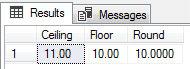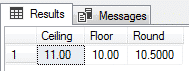Click on the banner to learn about and purchase my database training on Azure

# SQL Server - Rounding numbers according to ABNT NBR 5891

Views: 26.836 views

Hey guys!

In this article, I will share with you some tips and explanations about number rounding in SQL Server using ROUND, FLOOR, CEILING and also using a custom function to meet the definitions of the ABNT NBR 5891 standard.

## Introduction

Present in virtually all information systems, rounding functions are widely used to handle fractional and decimal numbers, especially currency (\$). Because of this importance, it is very important that the rounding rules are very well understood so that there are no inconsistencies when rounding is applied to numbers, especially in large amounts of amounts.

To meet these needs, SQL Server provides us with 3 rounding functions:

• FLOOR: Returns an integer, always rounded down, ie returns the integer part of the entered decimal number.
• CEILING: Returns an integer value, always rounding up, that is, the integer + 1 of the informed decimal number (if the decimal value is> 0)
• ROUND: Returns a decimal value, rounding according to the number of decimal places specified in the function. By default, if the decimal place is <= 4, it will be rounded down. If the decimal place is> = 5, it will be rounded up.

This behavior can be changed by the 3 function parameter, which, when entered the value 0, will perform the truncation of the data instead of rounding, ie 10.999 with 2 decimal places would be 10.99.

However, when we have a system that needs to follow the ABNT NBR 5891 standard, we have a problem because the above functions do not meet the standard criterion, which is defined as follows:

#### Rounding Rules

The rounding rules, following the ABNT NBR 5891 Standard, apply to decimal digits in the position following the number of decimal digits you wish to transform, ie if we have a number of 4, 5, 6, n decimal digits and we want to round to 2, these rounding rules will apply:

If the following decimal digits are smaller than 50, 500, 5000…, The previous one does not change.
If the following decimal digits are larger than 50, 500, 5000…, The former is increased by one.
If the following decimal digits are equal to 50, 500, 5000..., the previous one is verified; if it is even, the former does not change; if it is odd, the former is increased by one.

Examples
Rounding to 2 decimal digits we should take into account the third and fourth decimal. Thus, according to the previous rules:

The number 12,6529 would be rounded to 12,65 (here is 12.65, since 29 is less than 50, so it doesn't change)
The number 12,86512 would be rounded to 12,87 (here is 12.87, since 512 is greater than 500, so one unit increments)
The number 12,744623 would be rounded to 12,74 (here is 12.74, since 4623 is less than 5000, so it doesn't change)
The number 12,8752 would be rounded to 12,88 (here is 12.88, since 52 is greater than 50, so one unit increments)
The number 12,8150 would be rounded to 12,82 (here is 12.82, since the following digits is equal to 50 and the previous one is odd, in this case 1, so one unit is incremented)
The number 12,8050 would be rounded to 12,80 (here is 12.80, since the following digits equals 50 and the previous one is even, in this case 0, so the previous one doesn't change)
The 13,4 Number666…, If we were to round to the whole part, it will always be rounded to 13, because 4666… it will always be less than 5000… (If we do the rounding number by number, we would have: 13,4666… → 13,47 → 13,5 → 14, however, that would be to say that 13,4666 ... is closer to 14 than it is 13, which is not true. Therefore, we should not round the number previously rounded !!!)

Reference: https://en.wikipedia.org/wiki/Rounding

## Number rounding with FLOOR, ROUND and CEILING

As noted in the introduction to this article, SQL Server provides us with 3 rounding functions:

• FLOOR: Returns an integer, always rounded down, ie returns the integer part of the entered decimal number.
• CEILING: Returns an integer value, always rounding up, that is, the integer + 1 of the informed decimal number (if the decimal value is> 0)
• ROUND: Returns a decimal value, rounding according to the number of decimal places specified in the function. By default, if the decimal place is <= 4, it will be rounded down. If the decimal place is> = 5, it will be rounded up.

This behavior can be changed by the 3 function parameter, which, when entered the value 0, will perform the truncation of the data instead of rounding, ie 10.999 with 2 decimal places would be 10.99.

I will demonstrate some examples to make it easier to understand the difference of these functions:In this example, we will use the 3th parameter of the ROUND () function to force rounding up (0 = standard rounding, ie 0 to 4 rounds down and 5 to 9 rounds up) and truncation (1 = Truncating, ie does not round, simply cuts the decimal places above the limit specified in the function).As you can see, the ROUND () function meets 2 of the 3 rules defined in the ABNT standard, but in the example of the value 10.225, according to the standard, rounding should have been done for 10,22 and not 10,23.

## Number rounding following ABNT NBR 5891

As we saw in the previous examples, when we fell into the rule “If the following decimal numbers are equal to 50, 500, 5000…, the previous one is verified; if it is even, the former does not change; if it is odd, the former is increased by one. ”, SQL Server standard rounding functions do not meet this need.

For this, I will provide the function below, which can fully comply with the rounding definitions of the standard ABNT NBR 5891:

#### Examples of use

The number 12,6529 would be rounded to 12,65 (here is 12.65, since 29 is less than 50, so it doesn't change)The number 12,86512 would be rounded to 12,87 (here is 12.87, since 512 is greater than 500, so one unit increments)The number 12,744623 would be rounded to 12,74 (here is 12.74, since 4623 is less than 5000, so it doesn't change)The number 12,8752 would be rounded to 12,88 (here is 12.88, since 52 is greater than 50, so one unit increments)The number 12,8150 would be rounded to 12,82 (here is 12.82, since the following digits equals 50 and the previous one is odd, in this case 1, then one unit is incremented)The number 12,8050 would be rounded to 12,80 (here is 12.80, since the following digits equals 50 and the previous one is even, in this case 0, so the previous one does not change)Well, that's it, guys!
I hope you enjoyed this post and see you next time!